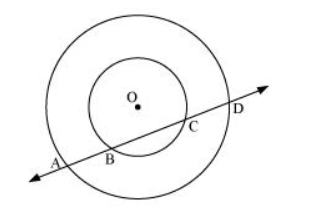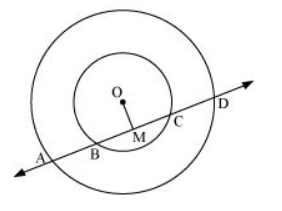# If a line intersects two concentric circles (circles with the same centre) with centre O at A, B, C and D

Question. If a line intersects two concentric circles (circles with the same centre) with centre O at A, B, C and D, prove that AB = CD (see figure 10.25).Solution:

Let us draw a perpendicular OM on line AD.It can be observed that BC is the chord of the smaller circle and AD is the chord of the bigger circle.

We know that perpendicular drawn from the centre of the circle bisects the chord.

$\therefore B M=M C \ldots(1)$

And, AM = MD ... (2)

On subtracting equation (2) from (1), we obtain

AM − BM = MD − MC

⇒ AB = CD